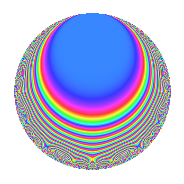Properties

 Label 50.2.dLevel 50 Weight 2 Character orbit d Rep. character $$\chi_{50}(11,\cdot)$$ Character field $$\Q(\zeta_{5})$$ Dimension 12 Newforms 2 Sturm bound 15 Trace bound 1

Related objects

Defining parameters

 Level: $$N$$ = $$50 = 2 \cdot 5^{2}$$ Weight: $$k$$ = $$2$$ Character orbit: $$[\chi]$$ = 50.d (of order $$5$$ and degree $$4$$) Character conductor: $$\operatorname{cond}(\chi)$$ = $$25$$ Character field: $$\Q(\zeta_{5})$$ Newforms: $$2$$ Sturm bound: $$15$$ Trace bound: $$1$$ Distinguishing $$T_p$$: $$3$$

Dimensions

The following table gives the dimensions of various subspaces of $$M_{2}(50, [\chi])$$.

Total New Old
Modular forms 36 12 24
Cusp forms 20 12 8
Eisenstein series 16 0 16

Trace form

 $$12q$$ $$\mathstrut -\mathstrut q^{2}$$ $$\mathstrut -\mathstrut 4q^{3}$$ $$\mathstrut -\mathstrut 3q^{4}$$ $$\mathstrut +\mathstrut 5q^{5}$$ $$\mathstrut -\mathstrut 2q^{6}$$ $$\mathstrut -\mathstrut 8q^{7}$$ $$\mathstrut -\mathstrut q^{8}$$ $$\mathstrut -\mathstrut 9q^{9}$$ $$\mathstrut +\mathstrut O(q^{10})$$ $$12q$$ $$\mathstrut -\mathstrut q^{2}$$ $$\mathstrut -\mathstrut 4q^{3}$$ $$\mathstrut -\mathstrut 3q^{4}$$ $$\mathstrut +\mathstrut 5q^{5}$$ $$\mathstrut -\mathstrut 2q^{6}$$ $$\mathstrut -\mathstrut 8q^{7}$$ $$\mathstrut -\mathstrut q^{8}$$ $$\mathstrut -\mathstrut 9q^{9}$$ $$\mathstrut -\mathstrut 5q^{10}$$ $$\mathstrut +\mathstrut 4q^{11}$$ $$\mathstrut +\mathstrut 6q^{12}$$ $$\mathstrut -\mathstrut 14q^{13}$$ $$\mathstrut -\mathstrut 4q^{14}$$ $$\mathstrut -\mathstrut 3q^{16}$$ $$\mathstrut -\mathstrut 8q^{17}$$ $$\mathstrut +\mathstrut 12q^{18}$$ $$\mathstrut +\mathstrut 10q^{19}$$ $$\mathstrut -\mathstrut 16q^{21}$$ $$\mathstrut +\mathstrut 8q^{22}$$ $$\mathstrut +\mathstrut 6q^{23}$$ $$\mathstrut +\mathstrut 8q^{24}$$ $$\mathstrut +\mathstrut 25q^{25}$$ $$\mathstrut +\mathstrut 18q^{26}$$ $$\mathstrut +\mathstrut 20q^{27}$$ $$\mathstrut +\mathstrut 2q^{28}$$ $$\mathstrut -\mathstrut 6q^{31}$$ $$\mathstrut +\mathstrut 4q^{32}$$ $$\mathstrut +\mathstrut 22q^{33}$$ $$\mathstrut +\mathstrut 11q^{34}$$ $$\mathstrut -\mathstrut 30q^{35}$$ $$\mathstrut -\mathstrut 9q^{36}$$ $$\mathstrut -\mathstrut 23q^{37}$$ $$\mathstrut -\mathstrut 20q^{38}$$ $$\mathstrut -\mathstrut 8q^{39}$$ $$\mathstrut -\mathstrut 5q^{40}$$ $$\mathstrut +\mathstrut 4q^{41}$$ $$\mathstrut -\mathstrut 22q^{42}$$ $$\mathstrut -\mathstrut 4q^{43}$$ $$\mathstrut -\mathstrut 6q^{44}$$ $$\mathstrut +\mathstrut 5q^{45}$$ $$\mathstrut +\mathstrut 8q^{46}$$ $$\mathstrut +\mathstrut 22q^{47}$$ $$\mathstrut +\mathstrut 6q^{48}$$ $$\mathstrut +\mathstrut 4q^{49}$$ $$\mathstrut -\mathstrut 25q^{50}$$ $$\mathstrut -\mathstrut 16q^{51}$$ $$\mathstrut -\mathstrut 14q^{52}$$ $$\mathstrut -\mathstrut 9q^{53}$$ $$\mathstrut -\mathstrut 20q^{54}$$ $$\mathstrut -\mathstrut 10q^{55}$$ $$\mathstrut -\mathstrut 4q^{56}$$ $$\mathstrut -\mathstrut 30q^{58}$$ $$\mathstrut +\mathstrut 4q^{61}$$ $$\mathstrut +\mathstrut 28q^{62}$$ $$\mathstrut +\mathstrut 16q^{63}$$ $$\mathstrut -\mathstrut 3q^{64}$$ $$\mathstrut -\mathstrut 15q^{65}$$ $$\mathstrut -\mathstrut 24q^{66}$$ $$\mathstrut +\mathstrut 2q^{67}$$ $$\mathstrut +\mathstrut 42q^{68}$$ $$\mathstrut +\mathstrut 52q^{69}$$ $$\mathstrut +\mathstrut 40q^{70}$$ $$\mathstrut -\mathstrut 6q^{71}$$ $$\mathstrut -\mathstrut 13q^{72}$$ $$\mathstrut +\mathstrut 6q^{73}$$ $$\mathstrut +\mathstrut 26q^{74}$$ $$\mathstrut +\mathstrut 50q^{75}$$ $$\mathstrut +\mathstrut 24q^{77}$$ $$\mathstrut +\mathstrut 24q^{78}$$ $$\mathstrut -\mathstrut 5q^{80}$$ $$\mathstrut -\mathstrut 33q^{81}$$ $$\mathstrut +\mathstrut 38q^{82}$$ $$\mathstrut +\mathstrut 26q^{83}$$ $$\mathstrut +\mathstrut 14q^{84}$$ $$\mathstrut +\mathstrut 35q^{85}$$ $$\mathstrut -\mathstrut 22q^{86}$$ $$\mathstrut -\mathstrut 2q^{88}$$ $$\mathstrut -\mathstrut 5q^{89}$$ $$\mathstrut +\mathstrut 25q^{90}$$ $$\mathstrut +\mathstrut 4q^{91}$$ $$\mathstrut +\mathstrut 6q^{92}$$ $$\mathstrut -\mathstrut 68q^{93}$$ $$\mathstrut -\mathstrut 24q^{94}$$ $$\mathstrut -\mathstrut 60q^{95}$$ $$\mathstrut -\mathstrut 2q^{96}$$ $$\mathstrut -\mathstrut 58q^{97}$$ $$\mathstrut -\mathstrut 17q^{98}$$ $$\mathstrut -\mathstrut 68q^{99}$$ $$\mathstrut +\mathstrut O(q^{100})$$

Decomposition of $$S_{2}^{\mathrm{new}}(50, [\chi])$$ into irreducible Hecke orbits

Label Dim. $$A$$ Field CM Traces $q$-expansion
$$a_2$$ $$a_3$$ $$a_5$$ $$a_7$$
50.2.d.a $$4$$ $$0.399$$ $$\Q(\zeta_{10})$$ None $$1$$ $$-1$$ $$5$$ $$-12$$ $$q+(1-\zeta_{10}+\zeta_{10}^{2}-\zeta_{10}^{3})q^{2}+(-1+\cdots)q^{3}+\cdots$$
50.2.d.b $$8$$ $$0.399$$ 8.0.58140625.2 None $$-2$$ $$-3$$ $$0$$ $$4$$ $$q+(-1+\beta _{2}+\beta _{3}+\beta _{6})q^{2}+\beta _{4}q^{3}+\cdots$$

Decomposition of $$S_{2}^{\mathrm{old}}(50, [\chi])$$ into lower level spaces

$$S_{2}^{\mathrm{old}}(50, [\chi]) \cong$$ $$S_{2}^{\mathrm{new}}(25, [\chi])$$$$^{\oplus 2}$$